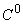Reports of Enlarged Session of the Seminar of I. Vekua Institute of Applied Mathematics

VOL 5,N3, 1990

volum dedicate to Professor Temury  Chanturias memory.

1.      Chanturia TA. Onthe oscilacions of linear high order differential equations . 6-8.

2.      Adiutov A.M., Klokov L.A. On the uniqueness of solution of the problem with conditions in infinity for the equation of nonlinear gazodynamic theory 9-12.

3.      Andrianov D.L. On the some of boundary problems of macromodellisising.13-16.

4.      Astashova I.V. On the some properties of solutions with alternating sings of one nonlinear differential equation.17-20.

5.      bogdanova M.Y., Kiguradze I.T. on the asymptotical estimation of Knezer’s solutions. 21-25.

6.      Brikalov S.A. Solutions of one boundary problem, aproximate this function.26-28.

7.      Bulgakov A.I. Functionasl-differential including with nonconvex right side.29-32.

8.      Bourmistrova A.V. Rezonance boundary problems for Functionasl-differential equations. 33-36.

9.      Vlasov V.V. On the some properties strong solutions of one class Functionasl-differential equations. 37-40.

10.  Gaprindashvili G.D. On existence bounded solutions and solutions with nnonincrizing norm of system nonlinear ordinary differential equations second order.41-44.

11.  Gegelia G.T. On the boundary problems periodical type for system of ordinary differential equations. 45-48.

12.  Giorgadze G.P. About oscillatoric  properties  of system ordinary differential equations with delayed argument. 49-53.

13.  Grizans G.P. On the one boundary problem for system of two second order differential equation. 54-57.

14.  Jgarkava D.T. Nesessery condition optimality for some controled objects, described with integral equations with mesure. 58-63.

15.  Domoshinski A.I. On one boundary problem, arising in ballistics theory. 64-67.

16.  Drakhlin M.E. On one nesessery conditions of functionals extremum. 68-71.

17.  Evtoukhov V.M. About question on asymptotical integrability of linear differential equations. 72-75.

18.  Egorov Y.V., Kondrat’ev V.A. On etimation of minimal eigen-value  of Sturm-Liuvill’s problem . 76-79.

19.  Zviagintsev A.I. On precise estimations for intermediate differentials.80-83.

20.  Kakabadze L.V. On the properties of parts of systems of eigen functions of elliptic differential beam. 84-88.

21.  Koplatadze R.G. On Knezer’s solutions n-th order differential equation with delaing. 89-93.

22.  Kostin A.V., Okara D.V. Aproximation O-solutions asymptotical constants quasilinear systems with truncated  summs of Liapunov’s series. 94-97.

23.  Kurbatov V.N. On one estimation of perturbations , when remain stability.98-101.

24.  Labovski S.M. On monoton solutions of linear functional-differential equation. 102-105.

25.  Lepin L.A. Set of nonmonoton solutions of one boundary problem from nonlinear heat conductivity theory.106-107.

26.  Magnaradze L.G. About  Coshi problem for nonlifshits class ordinary differential equations in Banakh’s space.108-113.

27.  Maiorov V.E. Integral multiplicative inequality and existace theorem for the high order singular differential equations. 114-117.

28.  Maksimov V.P. Remark on  the linear boundary problems with conditions in inequaliti forms.118-119.

29.  Mamii D.K. Singular points first order nonroughty , definned by differential equation second order witgh dicontinuiting  right side.120-124.

30.  Millionshikov V.M. On the orbital stability. 125.

31.  Mirzov D.D. About conditions of oscilation of solutions of one nonlinear system.126-129.

32.  Murad’ian M.G. On one invers problem, from displasement’s theory.130-133.

33.  Nadareishvili V.A. On monoton solutions of system of linear differential equations first order with delayed argument.134-138.

34.  Nikonenko V.V. On asymptotic represantation of solutions of linear system of ordinary differential equations. 139-142.

35.  Paatashvili D.V. On nonosciliatority  linear differential equations second order with delayed arguments. 143-147.

36.  Pilugin S.Y. Chain extended and-closing. 148-150.

37.  Rabimov A.S. On boundary problem with parameter. 151-155.

38.  Rabtsevich V.A. On monoton right solutions generalized Emden-Fauler’s equation. 156-159.

39.  Reizin L.E. Dynamical system with nononedimentional group. 160-163.

40.  Reinfeld A.A. Dynamical equivalency for dynamical extentions.164-166.

41.  Ronto N.I. On numerical-analitic method for boundary problems ordinary differential equations. 167-170.

42.  Samkova G.E. On existance bounded analitic solutions of differential systems.171-174.

43.  Sartabanov J.K. To methods of study multi-periodic solutions of D-equations systems. 175-178.

44.  Skhaliakho Ch.A. On zeros of sollutions of Emden-DFauler’s equations.179-182.

45.  Tadumadze T.A., Dvalishvili F.A. On existance of solutions and smooth dependence functional’s minimum in the problems of optimal control with  distributed delaing.183-187.

46.  Fedoriuk M.V. Sturm-Liuvill’s problem with characteristics.188-190.

47.  Khvedelidze N.N. On the oscilation any solutions of thirth order linear ordinary differentil equations. 191-194.

48.  Tsitskishvili R.A.  Uniqueness of solution and correctness of ninear problem for functional-differential  equation. 195-198.

49.  Tskhovrebadze G.D. On correctness of  weighted  Valle-Poussen’s problem.199-203.

50.  Chelkas L.A. On propeties of functions of limit cycles of quadratic system in plaene. 204-206.

51.  Chichua D.I. On non-increesing solutions of second order differentil equations with argument. 207-211.

52.  Shevelo V.N., Gritsai A.G. On some properties functions, defined with differentil equations with involutions.212-215.

53.  Shekhter B.L. On uniqueness of monoton solutions of some boundary problems. 216-218.

54.  Sukina I.S. Mathematical modeling of twodimentional process of termotreatment of composit materials with real stresses. 219-222.